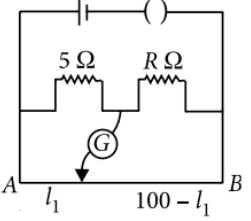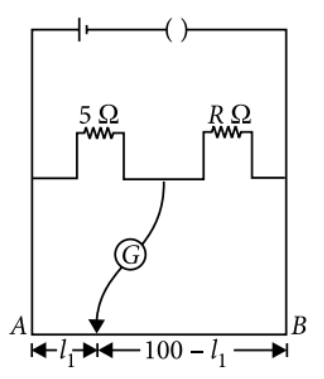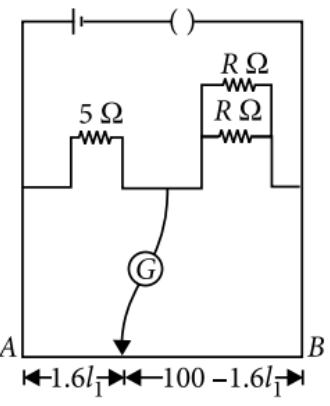meter bridge
Question

# The resistances in the two arms of the meter bridge are 5 $\Omega$ and R $\Omega$ respectively. When the resistance R is shunted with an equal resistance, the new balance point is at 1.6${\mathcal{l}}_{1}$.The resistance R isModerate
Solution

## In the first case,At the balance point$\frac{5}{R}=\frac{{l}_{1}}{100-l}$                              . . . . . (i)In the second caseAt the balance point$\frac{5}{\left(R/2\right)}=\frac{1.6{l}_{1}}{100-1.6{l}_{1}}$            . . . . . (ii)Divide eqn. (i) by eqn. (ii), we get $\frac{1}{2}=\frac{100-1.6{l}_{1}}{1.6\left(100-{l}_{1}\right)}$$160-1.6{l}_{1}=200-3.2{l}_{1}$Substituting this value in eqn. (i), we get $\frac{5}{R}=\frac{25}{75}$$R=\frac{375}{25}\Omega =15\Omega$

Get Instant Solutions